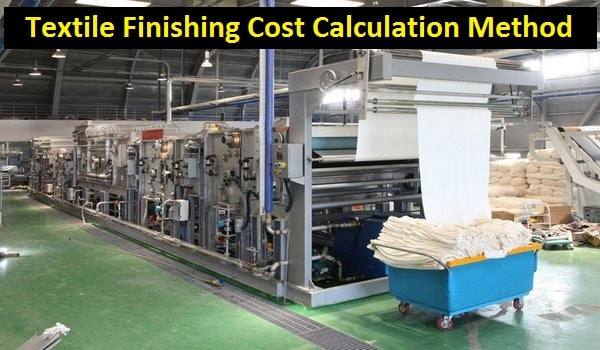# Apparel Costing

Solution of textile and apparel calculation

## How to Calculate Finishing Cost and Finishing Rate in Textile Industry?

Finishing Cost Calculation Process in Textile:
To change the fabric appearance, textile finishing process has great importance in textile industry. Finishing cost also included when total cost is calculated. So, to know the actual method of textile finishing cost calculation is mandatory for the responsible person.

You may follow:Fig: Textile finishing cost calculation method
Textile Finishing Cost Calculation Method:
Calculate the finishing cost and rate per of by following the below chart.
 SL No. Parameters Quantity and Cost 01 Fabric quantity 400kg 02 Finishing chemical consumed 5kg 03 Chemical A consumed 4kg 04 Chemical B consumed 2kg 05 1 day 3 shift 06 Total finishing process time 4 hours 07 Number of labors 3 08 Labor wages Rs. 200 per shift 09 Compacting Rs. 8 per kg 10 Steam Rs. 30000 per month 11 Power Rs. 10000 per month 12 Transport Rs. 20000 per month 13 Salary Rs. 50000 per month 14 Other expenses Rs. 40000 per month 15 Finishing chemical price Rs. 250 per kg 16 Chemical A price Rs. 25 per kg 17 Chemical B price Rs. 60 per kg 18 Profit 15%

Solution:
To calculate textile finishing cost and finishing rate, below formula has to apply.

Finishing cost per kg,

Direct labor + Direct material + Direct expense + Direct overhead
…………………………………………………………………....…………      .... (1)
Total finishing production

Total Finishing Production Calculation:

Total production,
= Fabric input
= 400kg

Total Finishing Production Time Calculation:

Total finishing production time,
= Finishing process time
= 4 hours
= (4/8) shift
= 0.5 shift

Direct Labor Cost Calculation:

Operator wages,
= Number of labor × No. of shift × labor wage per shift
= 3×0.5× Rs. 200
= Rs. 300

Direct Material Cost Calculation:

Finishing chemical cost = Amount of chemical consumed × chemical price = 5× Rs. 250 = Rs. 1250

Chemical A cost = Amount of chemical consumed × chemical price = 4× Rs. 25 = Rs. 100
Chemical B cost = Amount of chemical consumed × chemical price = 2× Rs. 60 = Rs. 120

So,
Direct material cost is,
= (Rs. 1250 + Rs. 100 + Rs. 120)
= Rs. 1470

Direct Expense Cost Calculation:

Compacting,
= Fabric quantity × compacting rate per kg
= 400 × Rs. 8
= Rs. 3200

In steam cost calculation,
= (Cost × 0.5 shift) / (30days × 3 shifts in a day) = {(30000 × 0.5)/90} = Rs. 167

In transport cost calculation,
= (Cost × 0.5 shift) / (30days × 3 shifts in a day) = {(20000 × 0.5)/90} = Rs. 112

In power cost calculation,
= (Cost × 0.5 shift) / (30days × 3 shifts in a day) = {(10000 × 0.5)/90} = Rs. 56

In other expenses cost calculation,
= (Cost × 0.5 shift) / (30days × 3 shifts in a day) = {(40000 × 0.5)/90} = Rs. 224

In salary cost calculation,
= (Cost × 0.5 shift) / (30days × 3 shifts in a day) = {(50000 × 0.5)/90} = Rs. 279

So,
= Rs. 167 + Rs. 112 + Rs. 56 + Rs. 224 + Rs. 279
= Rs. 838

Now, from equation-01, we get,

Textile finishing cost per kg,

(Rs. 1470 + Rs. 300 + Rs. 3200 + Rs. 838)
…………………………………………………………..
400

= Rs. 14.50

After adding 15% profit, textile finishing rate stands at
= {14.50/ (1-15%)}
= Rs. 17.10